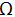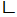# If Vab=43.9 < 200 and |Z|=√34 then which of the following represents Z

1.  3+j5

2.  3-j5

3.  -3-j5

4.  -3+j5

4

3+j5

Explanation :
No Explanation available for this question

# Both transistor has β0=125 and IC=1mA, RS=0.6K, RC=1.2 K. Find Av

1.  -1200

2.  -1400

3.  +1400

4.  +140

4

+1400

Explanation :
No Explanation available for this question

# If impedance between terminal A-B is 50.7

1.  5

2.  2

3.  10

4.  None of these

4

5Explanation :
No Explanation available for this question

# In an Intrinsic Si semiconductor effectively mass of electron is four times the effective mass of hole. Then What will be the position of Fermi level at T=270C

1.  0.55ev

2.  0.6ev

3.  0.52ev

4.  Data insufficient

4

0.52ev

Explanation :
No Explanation available for this question

# Consider the given statement: gm=(IC/VT)=( IC/25)mA

1.  Statement is correct

2.  Statement is correct but IC may not in mA

3.  Statement is false

4.  Statement is incomplete as equation holds on T=290k

4

Statement is incomplete as equation holds on T=290k

Explanation :
No Explanation available for this question

# If V0= (59/31) V, then X will be

1.  2A

2.  -2A

3.  1A

4.  -1A

4

2A

Explanation :
No Explanation available for this question

# consider a Si semiconductor whose ni-1.5*1010/cm-3 and. Its conductivity will be minimum when n0 will be equal to

1.  9*109/cm3

2.  9*1010/cm3

3.  10*1010/cm3

4.  data insufficient

4

9*109/cm3

Explanation :
No Explanation available for this question

# consider a Si semiconductor whose ni-1.5*1010/cm-3 and . At n0 its conductivity will be

1.  2*10-6(Ω-cm)-1

2.  3.9*10-6(Ω-cm)-1

3.  6*10-6(Ω-cm)-1

4.  None

4

3.9*10-6(Ω-cm)-1

Explanation :
No Explanation available for this question

# Current through the inductor is

1.  2-63.430

2.  2-73.70

3.  -273.70

4.  -2-73.70

4

2-63.430

Explanation :
No Explanation available for this question

# Diffusion current in a p-n junction is influenced by

1.  Applied voltage

3.  Concentration

4.  all of these

4